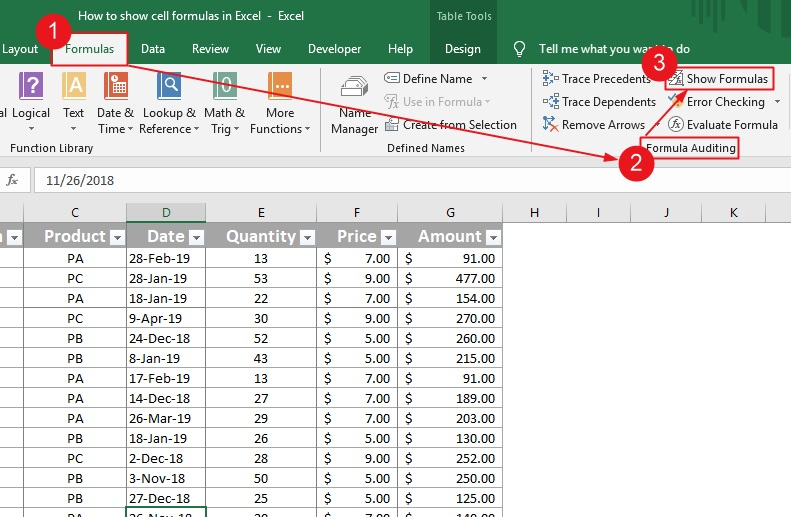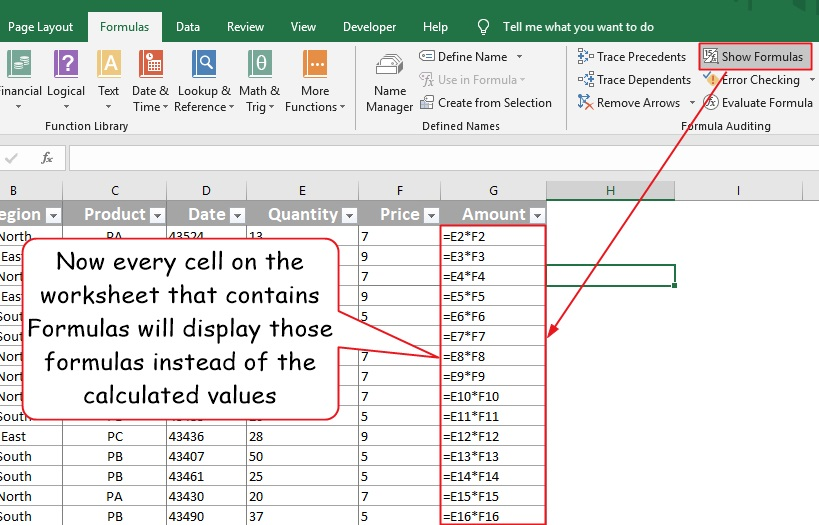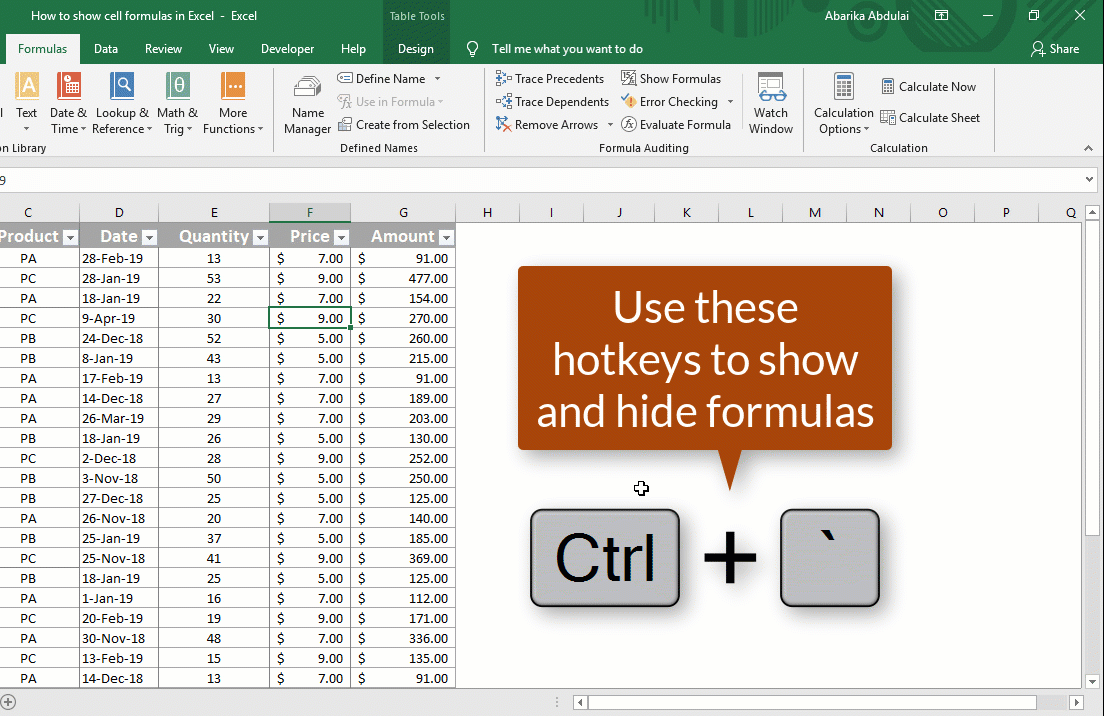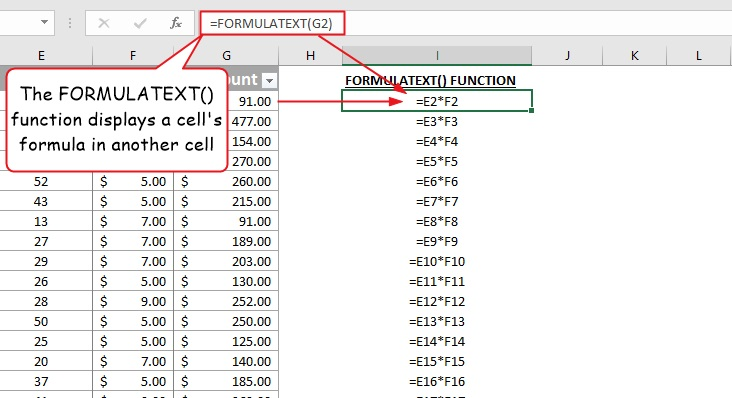How to show cell formulas in Excel is one of the frequently asked questions about formulas. As soon as you enter a formula in a cell, Excel shows the calculated values rather than the formula text.

This behavior is usually what you want to happen when you enter a formula into a cell. But in some cases, you may want to show the formulas that generated the results or even print those formulas instead of the calculated values.

You could go through each cell, one after the other, and look at the formula in the formula bar. You could also use the F2 or double click to see the formula right in the cell.

What if I tell you there is a technique to display all formulas in the worksheet at the same time? Let me quickly show you this technique.

• Go to Formulas→Formula Auditing→Show Formulas.At this point, every cell that contains a formula will display those formulas instead of the calculated values. The columns automatically widen to show more details since formulas tend to be longer than their results. But this affects the current worksheet only.

To hide formulas, just follow the same process and click show formula to turn it off.

See screenshot:## Show formula shortcut

The process above is somewhat a painstaking process of displaying cell formulas in Excel. Is there a faster way one could use instead? Yes, there is – the show formula shortcut.

On most of the U.S keyboards, just below the Esc key is a key with two characters – one is called the tilde and the other is called the grave accent.

• Hold down Ctrl + this key to toggle into show formula mode. Again, each column gets wider to allow you to see all of the formulas. Pressing these same keys will hide the formulas.

See screenshot:## Show formulas function in excel

By now I assume that you already know how to use the Show Formulas mode to show and hide Excel formulas.

There is a permanent technique to perform this task – i.e. using the FORMULATEXT() function.

Using this function, you can show formula in another cell by referencing to the cell that contains the formula. This function is only available in Excel 2013 and later.

See screenshot below:If your formulas are in a series of sells like in the one above, perform this function in the first cell and use the AutoFill handle to apply to the rest of the cells.

To do that, just click and drag the fill handle downwards to copy the formula to the rest of the cells.

However, you should use relative cell referencing for this trick to work.